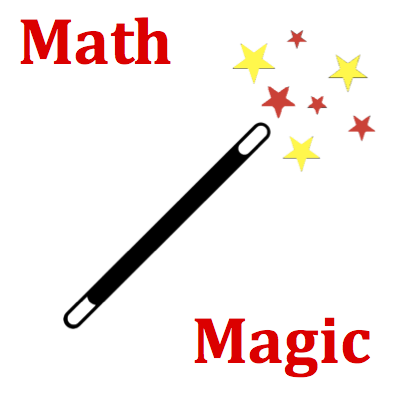# Circular and Satellite Motion - Mission CG2 Detailed HelpAn object with mass 'm' is moving in a circle of radius 'R' at a constant speed of 'v.' The net force acting upon the object must be INCREASED if ____. List all that apply ... .Equations are guides to assist you in thinking about relationships between variables. In this question, the relationship between mass, radius, velocity and acceleration is explored. The equations in the Formula Frenzy section below show the dependency of acceleration upon speed and radius and of net force upon mass and acceleration. Thinking about the equations in a conceptual manner allows you to answer this question. Clearly the acceleration is directly related to the speed and inversely related to the radius. A change in acceleration will affect the required net force in a direct manner. Finally, any change in mass will directly affect the net force as well.The acceleration (a) of an object that is moving in a circle is dependent upon the speed (v) at which it moves and the radius (R) of the circular path about which it moves. The relationship is expressed by the following equation: a = v2​​ / R   The net force (Fnet) required to cause such an acceleration is dependent upon the mass (m) of the object and the rate of acceleration (a). The relationship is expressed by the following equation. Fnet = m • a